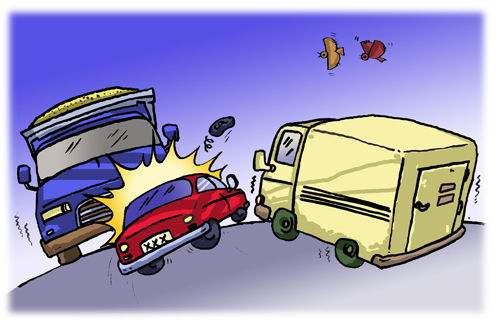Skip to main content
首页 » 保险案例

# 机动车保险理赔案例分析，保险理赔应该这样计算

案例：

李某驾驶的载货汽车(甲车)与王某驾驶的小型客车(乙车)相撞，两车负有同等责任，导致甲车车辆损失3000元，车上货物损失5000元;乙车车辆损失10000元，乙车车上一名人员重伤致残，医疗费用20000元，残疾赔偿金50000元。(此次事故还造成了骑自行车的张某重伤抢救无效死亡，产生医疗费用30000元，死亡赔偿金100000元，精神抚慰金20000元。路产损失5000元。考虑到很多朋友不太了解保险理赔，本次就不分析骑自行车张某和路产损失的理赔，下次再和大家分析。)

甲乙两车均投保了交强险;甲车投保了车辆损失险、第三者责任险、车上货物责任险、不计免赔险;一车投保车辆损失险、第三者责任险、车上人员责任险、不计免赔险。

理赔计算

思路：一般情况下，机动车辆都投保了好几个险种，但是总体可分为交强险和商业保险，要分开计算。交强险又分为才惨损失、医疗费用和死亡伤残赔偿。这三项赔款的限额前面已经和大家介绍过了。

赔偿限额种类有责任(元)无责任(元)

死亡伤残11000011000

理疗费用100001000

财产损失20001000

一、交强险赔款计算

1、甲车赔偿金额

(1)财产损失赔偿金额

受损财产核定金额=乙车车辆损失

=10000>2000

财产损失赔偿金额=2000元

其中

乙车得到赔偿=2000元

(2)医疗费用赔偿金额

医疗费用核定损失金额=20000>10000

医疗费用赔偿金额=10000元

其中

乙车人员得到赔偿=10000元

(3)死亡伤残费用赔偿金额

死亡伤残损失核定损失金额=50000<110000

死亡伤残赔偿金额=50000元

其中

乙车人员得到赔偿=50000元

汇总有

甲车交强险总赔偿金额=2000+10000+50000=62000

2、乙车赔偿金额

(1)财产损失赔偿金额

受损财产核定损失金额=甲车车辆损失+甲车车上货物损失

=3000+5000=8000>2000

财产损失赔偿金额=2000元

其中

甲车得到赔偿=2000元

汇总有

乙车交强险赔偿金额=2000元

本案例中各方分项得到的交强险赔偿

甲车得到

财产损失=2000元

其中

赔偿车辆=3000/(3000+5000)*2000=750元

赔偿货物=5000/(3000+5000)*2000=1250元

乙车得到

车辆损失赔偿=2000元

医疗费用赔偿=10000元

死亡伤残赔偿=50000元二、商业保险赔款计算

1、甲车

车辆损失赔偿金额=(车辆损失—交强险赔偿金额)*责任比例

=(3000—750)*50%=1125元

车上货物责任赔偿金额=(5000—1250)*50%=1875元

第三这责任险赔偿金额=(乙方损失-交强险赔偿金额)*50%

=(10000+20000+50000—2000—10000—50000)*50%

=9000元

甲车商业保险赔偿金额=1125+1875+9000=12000元

2、乙车

车辆损失赔偿金额=(10000—2000)*50%=4000元

车上人员责任保险赔偿金额=(20000+50000—10000—50000)*50%

=5000元

第三者责任险赔偿金额=(甲方损失—交强险赔偿金额)*50%

=(3000+5000—2000)*50%=3000元

乙车商业保险赔偿金额=4000+5000+3000=12000元

汇总整理全部计算结果：

甲乙两车交强险和商业保险全部赔偿金额=62000+2000+12000+12000

=88000元

所有参与方的损失金额=3000+5000+10000+20000+50000=88000元说明

由于保险是一种补偿性的业务，不会给投保人或者被保险人带来额外的利益，他要求赔款最终不能高于被保险标的的损失。在上述案例中也可以看出交强险和商业保险最终的赔款之和和各方面的损失金额是相等的，这可以验算保险理赔计算的是否正确。

可以看出，保险理赔计算式一个相当复杂的过程，上述案例在没有考虑其自行车的张某和路产损失的情况下，计算过程就已经领不少人头昏脑涨了。不过通过这一案例分析，大家至少知道保险理赔计算的思路和方法。